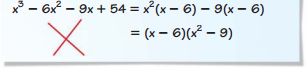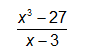#### MAT-HS.A-SSE.02

 MAT-HS Targeted Standards(A) Concept: Algebra(SSE) Domain: Seeing Structure in ExpressionsCluster: Interpret the structure of expressions MAT-HS.A-SSE.02 Use the structure of an expression to identify ways to rewrite it. For example, see x4 – y4 as (x²)² – (y²)², thus recognizing it as a difference of squares that can be factored as (x² – y²)(x² + y²).

## Student Learning Targets:

### Knowledge Targets

• I can recall the order of operations.
• I can understand and identify a power and its parts (base and exponent).
• I know the properties of exponents (i.e. product of powers, power of a power, power of a product).
• I can recognize a zero or negative exponent.
• I can recall the order of operations.
• I can understand and identify a power and its parts (base and exponent).
• I know the properties of exponents (i.e. product of powers, power of a power, power of a product).
• I can recognize a zero or negative exponent.

### Reasoning Targets

• I can analyze an algebraic expression.
• I can decide what to do next based on my knowledge of the order of operations.
• I can analyze an algebraic expression.
• I can decide what to do next based on my knowledge of the order of operations.

### Skills (Performance) Targets

• I can factor algebraic expressions.
• I can combine like terms to simplify an algebraic expression.
• I can perform basic operations.
• I can simplify algebraic expressions.
• I can apply order of operations.
• I can simplify an algebraic expression.
• I can rewrite algebraic expressions in equivalent forms such as factoring or combining like terms.
• I can use factoring techniques such as common factors, grouping, the difference of two squares, the sum or difference of two cubes, or a combination of methods to factor an expression completely.
• I can simplify expressions by combining like terms, using the distributive property and using other operations with polynomials.

• I can

## Proficiency Scale

 Score Description Sample Activity 4.0 In addition to achieving level 3.0 content, the student makes in-depth inferences and applications that go beyond what was taught. Factor the following algebraic expressions. x3+2x2-4x-8 6x3-14x2-12x 3.5 In addition to Score 3.0 performance, the student demonstrates in-depth inferences and applications regarding the more complex content with partial success. 3.0 The student: uses the structure of an expression to identify ways to rewrite it, using the following techniques to factor: factor by grouping. difference of perfect squares. greatest common factor. factor a trinomial. The student exhibits no major errors or omissions. Factor the following algebraic expressions. 4x2-8x+3 2x2-15x+18 3x2-5x-2 x3+8x2+6x+48 9x2-49 2.5 The student demonstrates no major errors or omissions regarding the simpler details and processes (Score 2.0 content) and partial knowledge of the more complex ideas and processes (Score 3.0 content). 2.0 There are no major errors or omissions regarding the simpler details and processes as the student: combines like terms to simplify an expression. (A.SSE.1) & (A.APR.1) uses the following techniques to factor: greatest common factor. factor a trinomial with a leading coefficient of 1. However, the student exhibits major errors or omissions regarding the more complex ideas and processes. Simplify the following algebraic expressions. 3x2+2x-6x2+4y+6+2x Factor the following algebraic expressions. 6x2+3x x2+4x+3 x2-3x-4 1.5 The student demonstrates partial knowledge of the simpler details and processes (Score 2.0 content) but exhibits major errors or omissions regarding the more complex ideas and procedures (Score 3.0 content). 1.0 With help, a partial understanding of some of the simpler details and processes and some of the more complex ideas and processes. - 0.5 With help, the student demonstrates a partial understanding of some of the simpler details and processes (Score 2.0 content) but not the more complex ideas and processes (Score 3.0 content).

## Alg II Equivalent Expressions Proficiency Scale

 Score Description Sample Activity 4.0 (advanced) In addition to Score 3.0, the student demonstrates in-depth inferences and applications regarding more complex material that go beyond end of instruction expectations. Describe the error in each of the following:3.5 In addition to Score 3.0 performance, the student demonstrates in-depth inferences and applications regarding the more complex content with partial success. 3.0 (proficient) The student can: recognize a suitable factoring technique given the structure of the expression. use factoring techniques such as common factors, grouping, the difference of two squares, the sum or difference of two cubes, or a combination of methods to factor an expression completely. rewrite algebraic expressions in equivalent forms such as factoring or combining like terms.   The student exhibits no major errors or omissions. Factor: x3+3x2-4x-12Factor and Simplify:2.5 The student demonstrates no major errors or omissions regarding the simpler details and processes (Score 2.0 content) and partial knowledge of the more complex ideas and processes (Score 3.0 content). 2.0 (progressing) There are no major errors or omissions regarding the simpler details and processes as the student can: simplify expressions by combining like terms, using the distributive property and using other operation with polynomials. understand that like terms can be combined using the distributive property and using other operations with polynomials. understand factoring techniques such as common factors, grouping, the difference of two squares, the sum or difference of two cubes, or a combination of methods to factor an expression completely.   recognize equivalent forms of the same expression.   However, the student exhibits major errors or omissions regarding the more complex ideas and processes. Factor: x2+5x-6  Factor and Simplify:1.5 The student demonstrates partial knowledge of the simpler details and processes (Score 2.0 content) but exhibits major errors or omissions regarding the more complex ideas and procedures (Score 3.0 content). 1.0 (beginning) With help, the student demonstrates a partial understanding of some of the simpler details and processes (Score 2.0 content) and some of the more complex ideas and processes (Score 3.0 content). 0.5 With help, the student demonstrates a partial understanding of some of the simpler details and processes (Score 2.0 content) but not the more complex ideas and processes (Score 3.0 content). 0.0 Even with help, the student demonstrates no understanding or skill.

## Resources

 Web Vocab